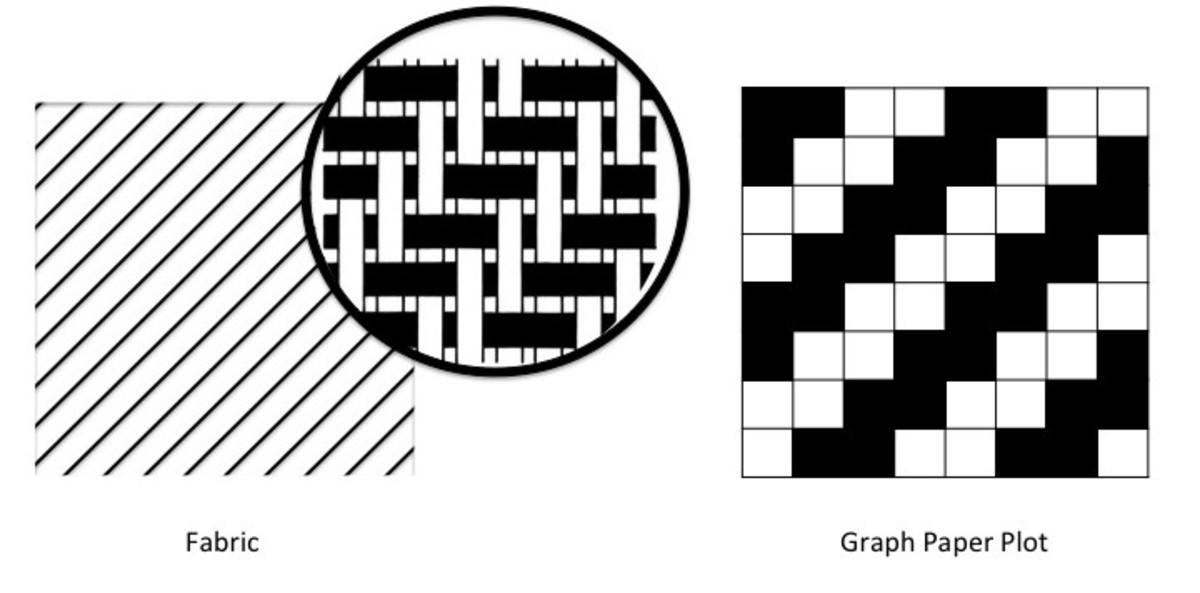# How to Analyze a Woven Fabric

I am a writer with special expertise in textiles, fabrics, printing, and dyeing.

## How Do You Analyze a Cloth or Woven Fabric?

A woven fabric is a fabric in which the yarns are arranged in an interlacement. The horizontal yarns (weft yarns) are interlaced with vertical yarns (warp yarns) to create a fabric.

There are mainly three ways to do an analysis of a woven fabric. There are many more methods, but these are the easiest for doing a quick fabric analysis. These are as follows:

• Analysis of the weave of the fabric
• Yarn count
• Yarns per inch (EPI/PPI)

*EPI stands for ends per inch

*PPI stands for picks per inch

## How to Do an Analysis of the Weave of the Fabric

To identify the arrangement of the interlacement of the yarns in the fabric, we follow the following steps to set the pattern on a graph paper. This examination is also referred to as weave analysis.

### Requirements for the Weave Analysis

• Fabric swatch
• Needle/pin
• Graph paper
• Magnifying glass/pick glass

### Weave Analysis, Step-by-Step

1. Determine which is the warp and which is the weft in the fabric. This can be easily identified by the selvage, which is always in the direction of the warp. The vertical would be the warp, and the horizontal would be the weft.
2. Place the fabric with its face upside and warp in the vertical direction.
3. Unravel a few yarns on the top edge and the right edge of the fabric swatch, to make a fringe of about 1 cm.
4. On the right edge, using a pin or a needle, unravel the first warp yarn slowly.
5. Using a magnifying glass, observe the interlacement of the warp over the weft from top to bottom of the fabric swatch.
6. On the graph paper, mark the following:
• Fill a square whenever the warp yarn is over the weft yarn.
• Leave the square blank when the warp yarn is under the weft yarn.
7. Repeat steps 1 to 6 to fill the graph paper, in order to reveal the interlacement arrangement.How to Do an Analysis of the Weave of the Fabric

## How to Calculate Yarn Count

According to the Textile Institute, “Count is a number which indicates the mass per unit length or the length per unit mass of yarn.”

### Requirements for the Calculation of Yarn Count Analysis

• Fabric swatch
• Pair of scissors
• Yarn count weighing machine
• Needle/pin

### Yarn Count Analysis, Step-by-Step

To calculate the yarn count, the following steps should be followed:

Scroll to Continue

1. Cut the fabric swatch into a size of 10 cm X 10 cm.
2. Take out 10 warp yarns from the fabric swatch using a needle. The total length of the yarns becomes 10 X 10 cm = 100 cm or 1 m.
3. Using a yarn count weighing machine, weigh the yarns.

If the weight of the yarns comes as x gm, the yarn count of warp yarn is (x/length in meter) X 1000 tex

Let’s say the weight of the yarns is 0.02 gm. The yarn count of the warp yarn will be:

= 0.02/1*1000

= 0.02*1000

= 20 tex

The same procedure should be followed to calculate the count of the weft yarns.

## How to Calculate Yarn Per Inch

This test gives us the construction of the fabric, i.e., in 1 inch X 1 inch area of fabric, how many warp and weft yarns are there.

### Requirements for the Calculation of Yarn Per Inch

• Fabric swatch
• Needle/pin
• Pick glass

### Calculate Yarn Per Inch, Step-by-Step

To calculate the yarns per inch, follow the following steps:

1. Place the fabric under a pick glass in a way the warp yarns align vertically to the side of the pick glass.
2. Using a pin or a needle, count the yarns that appear vertically in the fabric swatch, from left to right.
3. Similarly, using a pin or a needle, count the yarns that appear horizontally in the fabric swatch, from top to bottom.

If there are 100 warp yarns in an inch, the EPI would be 100. If there are 80 weft yarns in an inch, the PPI would be 80. The construction of the fabric would be EPI X PPI, i.e., 100 X 80.